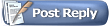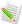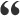Orbiter-ForumYet another propagation problem (atmospheric drag mostly)
 User Name Remember Me? Password
 Register Blogs Orbinauts List Social Groups FAQ Projects Mark Forums Read

 Math & Physics Mathematical and physical problems of space flight and astronomy.06-07-2018, 06:08 AM #16 Raccoon OrbinautQuote: Originally Posted by cristiapiDoes that code work? I read k2 = partialStep(y + k1 * h/2, ... what does y + k1 * h/2 mean? y is a Satellite structure or class; are you adding a vector to a class? This code work. y + k1 * h/2 mean your y[i] + stp * .5 * k1[i] Satellite is class, it has 2 vectors with length 3. First vector for location (loc) and second for velocity (vel). Quote: Originally Posted by cristiapiAlso, is not clear to me the use of RK4step(h, sat, Mjd_TT, T); (which is good) and dt = dt.addSecs(t); it should be dt = dt.addSecs(h) (even if it seems that you don't use dt). with RK4step(h, sat, Mjd_TT, T) I call RK4 method, I cant in good function names. Mjd_TT - it is modificated julian date for calculating atmosphere, T - is matrix which also need for calculating atmosphere. In example with only g= GM / r2 I dont comment it. Quote: Originally Posted by cristiapiThe function RK4step() is wrong. You should update a system of 2 differential equations, while you do a mix of RK4 with an Euler step. Here's my tested code for RK4: Code: ```void acc(VECTOR3 *y, VECTOR3 *f) { f = y; const double p= 1 / Vabs(y); f= y * -mu * p*p*p; } VECTOR3 k1, k2, k3, k4, yy; acc(y , k1); for(int i= 0; i < 2; i++) yy[i]= y[i] + stp * .5 * k1[i]; acc(yy, k2); for(int i= 0; i < 2; i++) yy[i]= y[i] + stp * .5 * k2[i]; acc(yy, k3); for(int i= 0; i < 2; i++) yy[i]= y[i] + stp * k3[i]; acc(yy, k4); for(int i= 0; i < 2; i++) y[i] += stp/6 * (k1[i] + 2 * k2[i] + 2 * k3[i] + k4[i]);``` where k* and y are positions and k* and y are velocities, stp is your h. I try your implementation of RK4: Code: ```void RK4step(const double& h, Satellite& y) { Satellite k1,k2,k3,k4, yy; acc(y , k1); //for(int i= 0; i < 2; i++) yy[i]= y[i] + stp * .5 * k1[i]; yy.loc = y.loc + h * .5 * k1.loc; yy.vel = y.vel + h * .5 * k1.vel; acc(yy, k2);//for(int i= 0; i < 2; i++) yy[i]= y[i] + stp * .5 * k2[i]; yy.loc = y.loc + h * .5 * k2.loc; yy.vel = y.vel + h * .5 * k2.vel; acc(yy, k3);//for(int i= 0; i < 2; i++) yy[i]= y[i] + stp * k3[i]; yy.loc = y.loc + h * .5 * k3.loc; yy.vel = y.vel + h * .5 * k3.vel; acc(yy, k4);//for(int i= 0; i < 2; i++) y[i] += stp/6 * (k1[i] + 2 * k2[i] + 2 * k3[i] + k4[i]); y.loc += h/6 * (k1.loc + 2 * k2.loc + 2 * k3.loc + k4.loc); y.vel += h/6 * (k1.vel + 2 * k2.vel + 2 * k3.vel + k4.vel); }``` Main loop Code: ``` double h = 1.0; for (int j = 0.0; j < 100; j++) { RK4step(h, sat); dt = dt.addSecs(h); // R3 - Earth radius = 6378.137 height.push_back(Magnitude2(sat.loc)-R3); satellite.push_back(sat.loc); satellite.push_back(sat.vel); }``` Function acc Code: ```void acc(Satellite &y, Satellite &f) { f.loc = y.vel; const double p= 1 / Magnitude2(y.loc); f.vel = y.loc * -MU * p*p*p; }``` But with your implementation height is rising from 639.69968632004 to 656.9692663242965. And with h = 0.1 from 639.6974238091 to 639.87063912038.06-08-2018, 08:50 PM #17 cristiapi OrbinautYour result is still wrong. Try the following test case (m, m/s): position: 7000e3, 0, 500e3 velocity: 0, 9e3, 0 GM= 398600.5e9 m3/s2 After 1000 s, the exact (analytical) result is: position: 3638361.4838039 7626460.47590472 259882.963128851 velocity: -5678.76636749567 5412.08256355282 -405.626169106835 If you use my RK4 code with h= 10 s, you must get: position: 3638361.48332985 7626460.47483667 259882.963094989 velocity: -5678.76636905365 5412.08256262949 -405.626169218118 if you get a different result, your implementation doesn't work.06-09-2018, 06:42 PM #18 Raccoon OrbinautQuote: Originally Posted by cristiapiIf you use my RK4 code with h= 10 s, you must get: position: 3638361.48332985 7626460.47483667 259882.963094989 velocity: -5678.76636905365 5412.08256262949 -405.626169218118 My result with meters and second and with h=10s. position: 3642902 7633650 260207.266 velocity: -5679.18213 5420.66357 -405.655853 Position without real part because QVector hold float type not double. So they are same as yours (not to much difference). I guess my calculation of height is wrong. Code: ```result = sqrt(x*x + y*y + z*z); // R3 - Earth radius = 6378.137 height = result - R3;``` Is it another methods to find height?06-10-2018, 09:32 PM #19 cristiapi OrbinautFloat? Consider that I wrote a 128-bit floating point numbers version for my integrator because the double type is not adequate for some applications. I strongly discourage you from using the float type, but if you really want to use it, you should get: position: 3638361.5 7626460 259882.90625 velocity: -5678.76806640625 5412.08154296875 -405.626190185547 Probably you should check again your implementation.06-11-2018, 05:54 PM #20 Raccoon OrbinautI dont need result be absolutly the same. I am ok with float. I just want to know why atmospheric drag doesnt work alone. And also how correclty calculate heigh?06-11-2018, 06:25 PM #21 cristiapi OrbinautIf you don't need accuracy, your Code: ```result = sqrt(x*x + y*y + z*z); // R3 - Earth radius = 6378.137 height = result - R3;``` is good.06-11-2018, 07:24 PM #22 Raccoon Orbinautso if my calculating formula for height is good. Why with this results height is rising?06-11-2018, 09:05 PM #23 cristiapi OrbinautBecause the satellite is in a point of the orbit where it goes from the perigee to apogee, the orbit is highly elliptical and the atmospheric drag is very small. In the long run, the orbit will decay (see my graph). Last edited by cristiapi; 06-11-2018 at 09:07 PM.06-13-2018, 04:01 PM #24 Raccoon OrbinautI understand what you mean. With only "g" height changes. But with atmospheric drag its only rising, how long must be "run"? Your graph shows only decreasing, it is solo atmospheric drag?06-13-2018, 08:15 PM #25 cristiapi OrbinautQuote: Originally Posted by RaccoonI understand what you mean. With only "g" height changes. But with atmospheric drag its only rising, how long must be "run"? Not sure to understand. Quote: Originally Posted by RaccoonYour graph shows only decreasing, it is solo atmospheric drag? Yes.06-15-2018, 02:20 PM #26 Raccoon OrbinautWith RK4 implementation of atmospheric drag will be like this? Code: ```void acc(Satellite &y, Satellite &f, double Mjd_TT,const QMatrix3x3 &T) { f.loc = y.vel; double Cd = 2.2; f.vel = AccelDrag(Mjd_TT, y.loc, y.vel, T, y.size, y.mass, Cd); } void RK4step(const double& h, Satellite& y, const double &Mjd_TT, const QMatrix3x3 &T) { Satellite k1,k2,k3,k4; Satellite yy; acc(y , k1, Mjd_TT, T); //for(int i= 0; i < 2; i++) yy[i]= y[i] + stp * .5 * k1[i]; yy.loc = y.loc + h * .5 * k1.loc; yy.vel = y.vel + h * .5 * k1.vel; acc(yy, k2, Mjd_TT, T);//for(int i= 0; i < 2; i++) yy[i]= y[i] + stp * .5 * k2[i]; yy.loc = y.loc + h * .5 * k2.loc; yy.vel = y.vel + h * .5 * k2.vel; acc(yy, k3, Mjd_TT, T);//for(int i= 0; i < 2; i++) yy[i]= y[i] + stp * k3[i]; yy.loc = y.loc + h * .5 * k3.loc; yy.vel = y.vel + h * .5 * k3.vel; acc(yy, k4, Mjd_TT, T);//for(int i= 0; i < 2; i++) y[i] += stp/6 * (k1[i] + 2 * k2[i] + 2 * k3[i] + k4[i]); y.loc += h/6 * (k1.loc + 2 * k2.loc + 2 * k3.loc + k4.loc); y.vel += h/6 * (k1.vel + 2 * k2.vel + 2 * k3.vel + k4.vel); }``` and main loop is: Code: ```Satellite sat(QVector3D(7000000.0, 0.0, 500000.0), QVector3D(0.0, 9000.0, 0.0), 62.5, 8000.0); QVector< QVector3D > satellite; double Mjd_UTC; double Mjd_TT; QVector height; double h = 10.0; QDateTime dt = QDateTime(QDate(2002,4,24),QTime(0,0,0,0)); for (int j = 0.0; j < 5000; j++) { Mjd_UTC = MJD(dt); Mjd_TT = Mjd_UTC + (32.0 + 32.184)/86400.0; QMatrix3x3 T = NutationMatrix(Mjd_TT) * PreccessionMatrix(MJD_J2000, Mjd_TT); RK4step(h, sat, Mjd_TT, T); dt = dt.addSecs(h); // R3 - Earth radius = 6378.137 height.push_back(Magnitude2(sat.loc) - R3*1000.0); satellite.push_back(sat.loc); satellite.push_back(sat.vel); }```06-18-2018, 10:16 AM #27 cristiapi OrbinautI see an error here: Quote: Originally Posted by RaccoonCode: ``` acc(yy, k3, Mjd_TT, T);//for(int i= 0; i < 2; i++) yy[i]= y[i] + stp * k3[i]; yy.loc = y.loc + h * .5 * k3.loc; yy.vel = y.vel + h * .5 * k3.vel;``` where do you see ".5"? Anyway, my sample code is not good for you because you need the full version with the time: https://en.wikipedia.org/wiki/Runge%...93Kutta_method notice f(tn + h/2,...). You could try to implement that code, but you should first understand what that code does.06-19-2018, 03:09 PM #28 Raccoon OrbinautI fix error. Solo atmospheric drag acceleration: Code: ```Satellite acc(double const &t, Satellite &y) { Satellite f; f.loc = y.vel; double Cd = 2.2; QMatrix3x3 T = NutationMatrix(t) * PreccessionMatrix(MJD_J2000, t); f.vel = AccelDrag(t, y.loc, y.vel, T, y, y.size, y.mass, Cd); return f; }``` Runge kutta with time will be: Code: ```void RK4step(const double& h, Satellite& y, double &t){ Satellite k1,k2,k3,k4, acceleration; Satellite yy; k1 = acc(t, y) * h; //for(int i= 0; i < 2; i++) yy[i]= y[i] + stp * .5 * k1[i]; yy.loc = (y.loc + .5 * k1.loc); yy.vel = (y.vel + .5 * k1.vel); yy.mass = y.mass; yy.size = y.size; k2 = acc(t + 0.5 * h, yy) * h; //for(int i= 0; i < 2; i++) yy[i]= y[i] + stp * .5 * k2[i]; yy.loc = y.loc + h * .5 * k2.loc; yy.vel = y.vel + h * .5 * k2.vel; k3 = acc(t + 0.5 * h, yy) * h; //for(int i= 0; i < 2; i++) yy[i]= y[i] + stp * k3[i]; yy.loc = y.loc + h * k3.loc; yy.vel = y.vel + h * k3.vel; k4 = acc(t + h, yy) * h; //for(int i= 0; i < 2; i++) y[i] += stp/6 * (k1[i] + 2 * k2[i] + 2 * k3[i] + k4[i]); y.loc += h/6 * (k1.loc + 2 * k2.loc + 2 * k3.loc + k4.loc); y.vel += h/6 * (k1.vel + 2 * k2.vel + 2 * k3.vel + k4.vel); }``` Main loop: Code: ``` double Mjd_UTC; double Mjd_TT; QVector height; double h = 10.0; QDateTime dt = QDateTime(QDate(2002,4,24),QTime(0,0,0,0)); for (int j = 0.0; j < 100; j++) { Mjd_UTC = MJD(dt); Mjd_TT = Mjd_UTC + (32.0 + 32.184)/86400.0; RK4step(h, sat, Mjd_TT); dt = dt.addSecs(h); // R3 - Earth radius = 6378.137 height.push_back(Magnitude2(sat.loc) - R3*1000.0); satellite.push_back(sat.loc); satellite.push_back(sat.vel); }``` Do I need send to RK modificated julian date (Mjd) or just send seconds starting, for example, from 0? What atmospheric model do you use? If its not hard can you post your implementation of solo atmospherid drag acceleration? I guess i dont calculate it right.06-20-2018, 09:02 AM #29 cristiapi OrbinautYour are still mixing code because you don't understand what RK4 does. Stop that random coding and take your time to understand RK4 without asking someone else to debug your random code.06-21-2018, 06:45 PM #30 Raccoon OrbinautHm, RK find value between to point (interpolating) to get close to real result. Higher rank then higher accuracy. And my code not random, i guess you dont understand what I ask. I dont ask anyone to debug my code, i want explanation how to calculate solo perturbed effects.Tags atmospheric drag, c++, propagationThread ToolsShow Printable VersionEmail this PagePosting Rules BB code is On Smilies are On [IMG] code is On HTML code is Off You may not post new threads You may not post replies You may not post attachments You may not edit your posts
 Forum Jump User Control Panel Private Messages Subscriptions Who's Online Search Forums Forums Home Orbiter-Forum.com     Announcements     Meets & Greets Orbiter Space Flight Simulator     Orbiter Web Forum         OFMM         Orbiter Forum Space Station         Simpit Forum     General Questions & Help     MFD Questions & Help     Hardware & Software Help     Tutorials & Challenges     Orbiter SDK     Orbiter Visualization Project     Orbiter Beta » Orbiter Project Orbiter Addons     OrbitHangar Addons & Comments     Addons     Addon Development     Addon Requests     Addon Support & Bugs         Addon Developer Forums             Project Apollo - NASSP     Orbiter Lua Scripting Far Side of the Moon     Spaceflight News     Math & Physics     Astronomy & the Night Sky     Backyard Rocketry     Brighton Lounge     International Forum

All times are GMT. The time now is 01:54 PM.

About Us | Rules & Guidelines | TOS Policy | Privacy Policy

Orbiter-Forum is hosted at Orbithangar.com